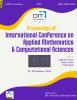# Study of Nonlinear Time Fractional Generalized Burger Equation with Proportional Delay via q-HAM

## Authors

Brajesh Kumar Singh
School of Physical and Decision Sciences, Department of Mathematics, Babasaheb Bhimrao Ambedkar University Lucknow-226025 (UP), INDIA
Saloni Agrawal
School of Physical and Decision Sciences, Department of Mathematics, Babasaheb Bhimrao Ambedkar University Lucknow-226025 (UP), INDIA

### Synopsis

The $$q$$-homotopy analysis method has been successfully implemented to study the nonlinear time-fractional generalized Burger equation with proportional delay (in brief, $$_{TF}GBE^{PD}$$), considering fractional derivative of Caputo type. The computed results are analyzed in terms of absolute error (and relative error norms in the fourth order approximation) for two test problems of nonlinear $$_{TF}GBE^{PD}$$. The relative error is reported for $$\alpha=0.8,0.9, 1$$ for different values of $$x,t \in [0, 1].$$ To guarantee the convergence of the results, $$\hbar$$-curves are depicted graphically for different values of the fractional order ($$\alpha$$). The findings shows that the proposed results are much better than the existing results and approaches towards the exact solution.Published
August 8, 2020
Series
Online ISSN
2582-3922